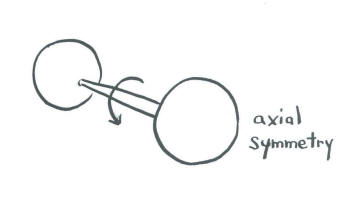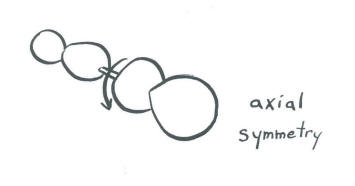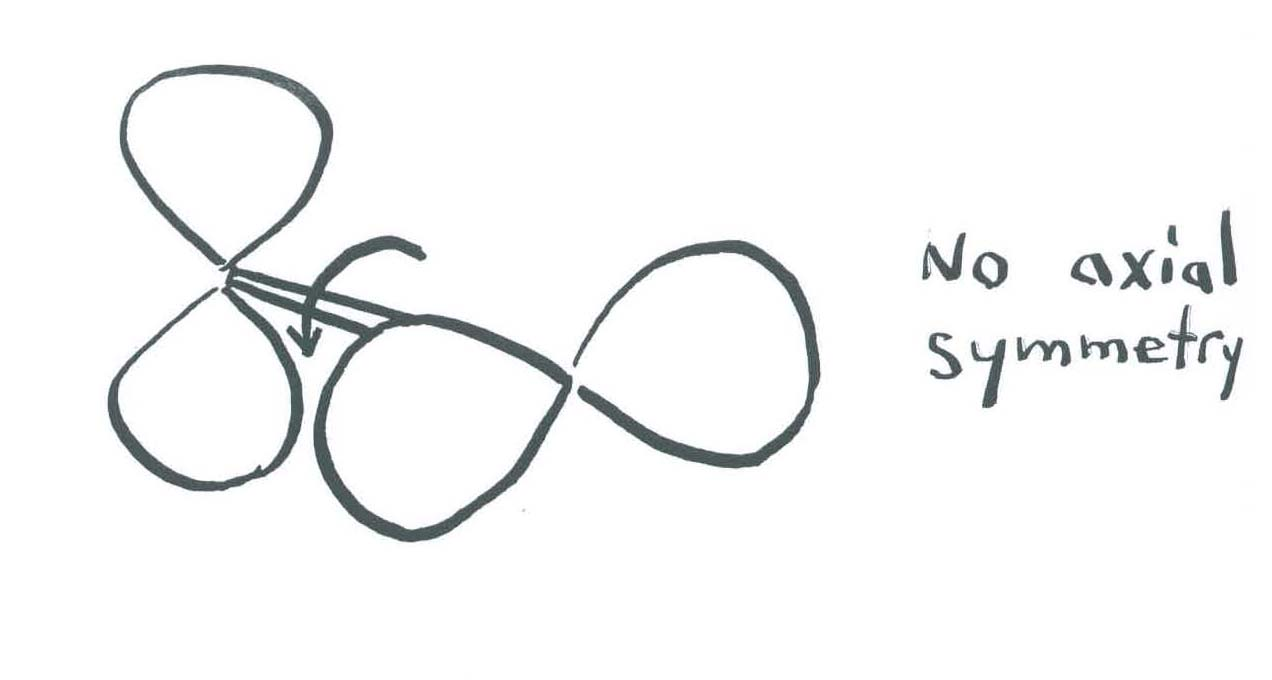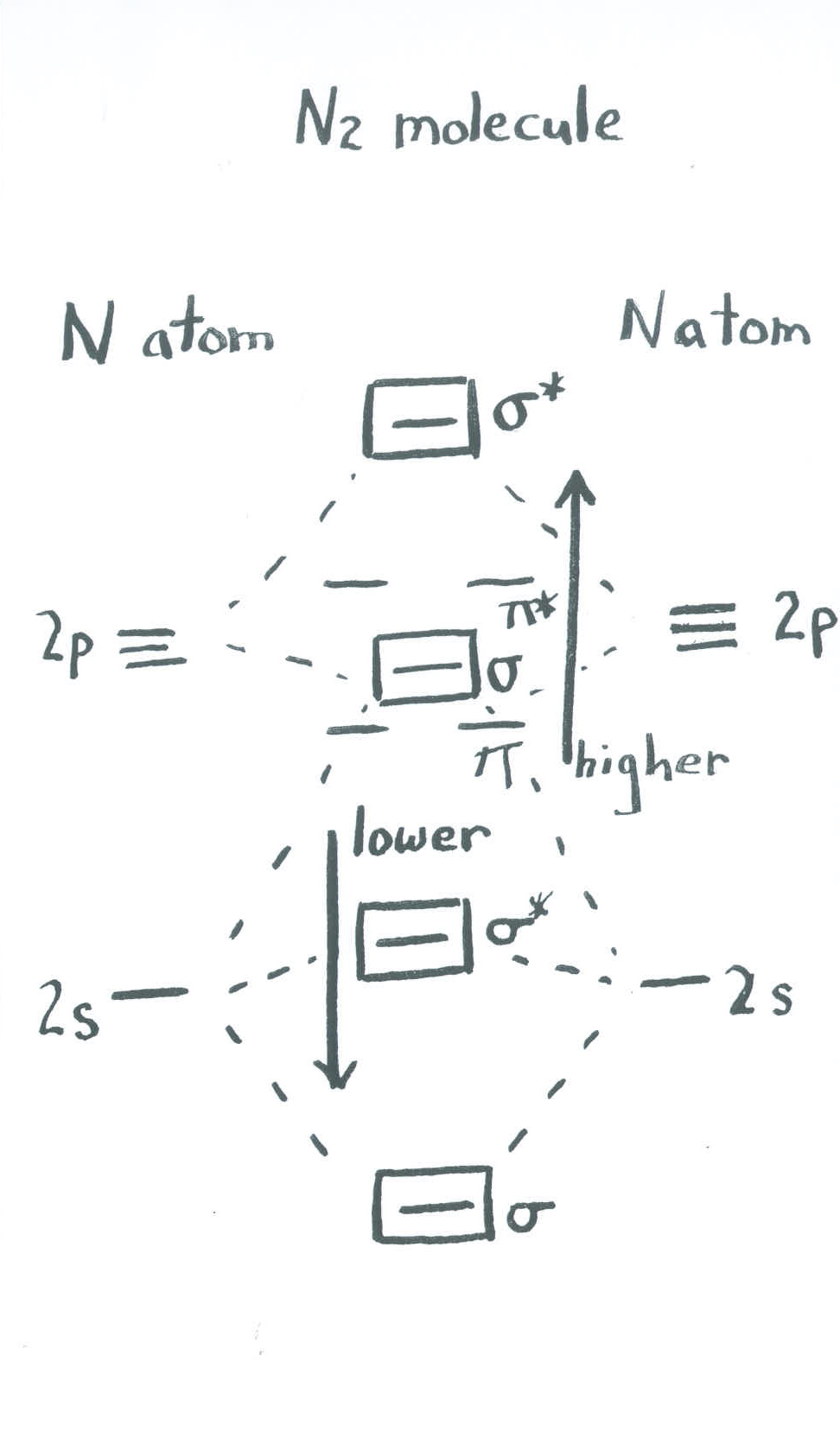# MO8. Symmetry & Mixing

### Symmetry and Mixing

At first, we approximated and said that only orbitals of the same kind can interact with each other: s orbitals with s orbitals, px orbitals with px orbitals, and so on. One of the qualitative differences between a real MO calculation and our scrap-paper approach is that mixing does occur between different kinds of orbitals. However, restrictions still apply.

Orbital interactions are governed by symmetry. Without taking an entire course in Group Theory (the branch of mathematics that deals with symmetry), we can learn a little bit about symmetry as we need it.

One of the most basic aspects of molecular symmetry applies to diatomic molecules. That element is symmetry about the bond axis. If a molecule is spun around its bond axis -- as if the two atoms are the two wheels on an axle of a wagon or a car -- does the orbital change its orientation?

• An s orbital is so symmetric that it looks the same from any direction. No matter how you spin it, it looks the same. In a diatomic molecule, an s orbital is symmetric with respect to rotation about the bond axis.• What about p orbitals? Suppose the bond axis is the z axis. Rotation about the z axis produces no change in orientation of a pz orbital. The orbital spins but does not move through space.
• Rotation about the z axis does produce a visible change in orientation of a px orbital. The orbital spins like a propeller around the axis.• Rotation about the z axis does produce a visible change in orientation of a py orbital. The orbital spins like a propeller around the axis.• As a result, in diatomics, an s orbital shares an element of symmetry with one p orbital, but not with the others.
• The rules of mathematics and quantum mechanics allow s orbitals in diatomics to interact with one p orbital with which it shares symmetry, but not with the other two.

Our picture of main group diatomics changes slightly with this news. The p bonding orbitals remain unchanged. However, the s bonding orbitals share axial symmetry. That means that the s bonding orbitals, far from keeping completely separate from each other, actually mix together in some cases. That means there can be an orbital that is composed of an s and pz orbital from one atom as well as an s and pz orbital from the other. The only restriction is that, because four atomic orbitals are allowed to mix, four new molecular orbitals must be produced.Another way of thinking about this mixing effect is to take the molecular orbital picture we already made for dinitrogen and then say that the sigma bonding orbitals can interact with each other. That means they can push each other up or down in energy, mixing together to create new combinations with new energies. The extent of this mixing varies across the periodic table.

For main group diatomics like N2, the effect is to lower the energy of the lower sigma orbitals (related to the atomic s levels) and raise the energy of the higher sigma orbitals (related to the atomic p levels). The result is that the second-highest sigma orbital is shifted slightly above the energy of the pi levels, rather than being slightly below them. This does not change the overall conclusions very much, but it is a favourable interaction because the lower-lying sigma levels, which are occupied by electrons, move to lower energy.